## Simple Matching Coefficient

Simple matching coefficient and Simple matching distance are useful when both positive and negative values carried equal information (symmetry). For example, gender (male and female) has symmetry attribute because number of male and female give equal information.

Formula: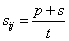Where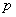= number of variables that positive for both objects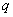= number of variables that positive for the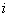th objects and negative for the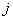th object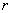= number of variables that negative for theth objects and positive for theth object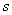= number of variables that negative for both objects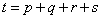= total number of variables

To measure the distance based on the simple matching coefficient, we have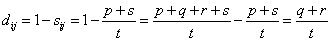Thus,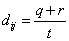Below is an interactive program to compute simple matching distance and simple matching coefficient. Try to experiment with your own input values.

Input coordinate values of Object-A and Object-B (the coordinate are binary, 0 or 1), then press "Get Simple Matching Coefficient" button to get Simple Matching distance and Simple Matching Coefficient.

Features Object A Object B

For example:

 Feature of Fruit Sphere shape Sweet Sour Crunchy Object=Apple Yes Yes Yes Yes Object=Banana No Yes No No

The coordinate of Apple is (1,1,1,1) and coordinate of Banana is (0,1,0,0). Because each object is represented by 4 variables, we say that these objects has 4 dimensions.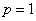,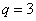and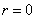,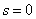.

In this example, the Simple Matching Coefficient (SMC) between Apple and Banana is 1/4. Simple Matching Distance (SMD) between Apple and Banana is 3/4.

Note that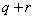is the Hamming distance .